GeeksforGeeks App
Open AppBrowser
Continue

# Capacitors in Series and in Parallel

The electrostatic potential is defined as the work done per unit charge. In more detail, the electric potential at any point in the electric field can be defined as the work done per unit charge in bringing the unit positive charge from infinity to that point, Infinity being the point outside the electric field. Work is required since the charge producing electric field applies a force on the unit positive charge after entering the field. Capacitance and electric potential are related in such a way that it is defined as the ratio of the electric charge (Q) to the electric potential (V) present in the conductor.

Formula for Electrostatic Potential:

V = W/q (volts)

Formula for Capacitance:

### Capacitors and Capacitance

Capacitors also known as condensers are the electrical devices used to store electric charge in order to store electrical energy, a capacitor is nothing but conductors placed at a certain distance “d” parallel to each other, the space between the conductors can either be vacuum or some insulating material/dielectric. The ability of the charge storage in a condenser or capacitor is known as Capacitance. Several times, multiple capacitors are connected in different combinations to achieve different levels of capacitance. These combinations can be arbitrarily complex, but they can be divided into two basic types:

1. Series Combination
2. Parallel Combination

Series Combination

In the figure given below, three capacitors are connected in series with the battery of voltage V. Note that in the figure, opposite charges of equal magnitude flow and get accumulated on the plates of the capacitor. Conservation of charge principles requires that the charge that is accumulated on the plates of the capacitor must be equal in magnitude. The end result is a combination that resembles a single capacitor with an effective plate separation that is greater than that of individual capacitors, this equivalent capacitor is shown in the figure below. Large plate separation means smaller capacitance.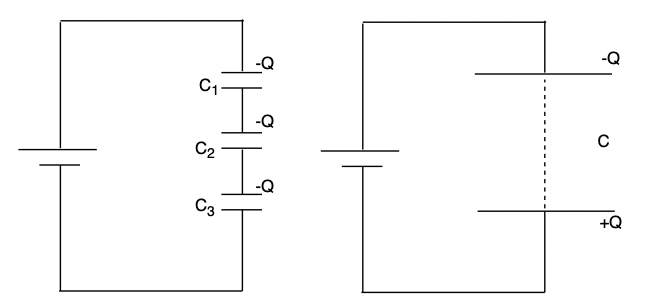The relation for capacitance is given by,

C = Q/V

It can be rewritten as,

V = Q/C

The voltages across individual capacitors will be,

V1 = Q/C1 , V2 = Q/C2, V3 = Q/C3

The total voltage across all the capacitors will be,

V = V1 + V2 + V3

substituting the expressions for individual voltages,

V = Q/C1 +Q/C + Q/C3

Let the equivalent capacitance be C,

Q/C = Q/C1 +Q/C + Q/C3

Upon Simplifying the above equation, the relation becomes,In general for capacitors C1, C2, C3, ….Parallel Combination

In the figure given below, three capacitors C1, C2, C3 are connected in parallel to a voltage source of potential V. Deriving the equivalent capacitance for this case is relatively simple. Note that the voltage across each capacitor is the same as that of the source since it is directly connected to the source. Thus capacitors have the same charges on them as they would have if connected individually to the voltage source. Let’s say the total charge on the capacitors is Q,

Q = Q1 + Q2 + Q3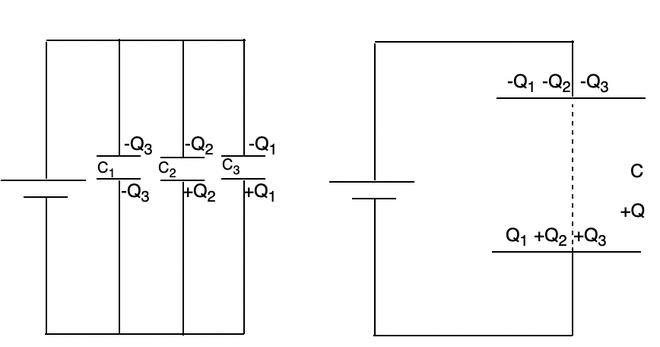Substituting the charges with their relation with capacitances.

Q = Q1 + Q2 + Q3

⇒ CV = C1V + C2V + C3V

⇒ C = C1 + C2 + C3

### Sample Problems

Question 1: Three capacitors of 3pF, 5pF, and 10pF are connected in parallel. Find the equivalent capacitance for the system.

The formula for series capacitance is given by,

C = C1 + C2 + C3

Given: C1 = 3pF, C = 5pF and C3 = 10pF

Substituting these values in the equation,

C = C1 + C2 + C3

⇒ C = 3 + 5 + 10

⇒ C = 18pF

Question 2: Three capacitors of 2pF, 2pF, and 4pF are connected in series. Find the equivalent capacitance for the system.

The formula for parallel capacitance is given by,Given: C1 = 2pF, C = 2pF and C3 = 4pF

substituting these values in the equation,⇒⇒⇒Question 3: Find the equivalent capacitance for the system shown in the figure below.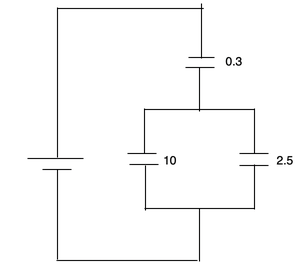The formula for parallel capacitance is given by,and the formula for series capacitance is given by,

C = C1 + C2 + C + ….

This is combination of both parallel and series capacitances.

Substituting these values in the equation,

C1 = 10 μF, C = 2.5 μF

C = C1 + C2

⇒ C = 10 + 2.5

⇒ C = 12.5⇒⇒⇒ C = 0.29

Question 4: Find the equivalent capacitance for the system shown in the figure below.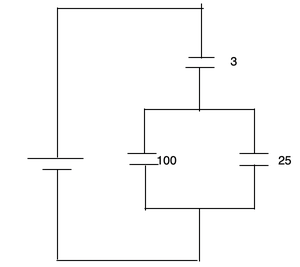The formula for parallel capacitance is given by,and the formula for series capacitance is given by,

C = C1 + C2 + C + ….

This is combination of both parallel and series capacitances.

substituting these values in the equation,

C1 = 100 μF, C = 25 μF

C = C1 + C2

⇒ C = 100 + 25

⇒ C = 125⇒⇒⇒ C = 2.9

Question 5: Find the equivalent capacitance for the system shown in the figure below.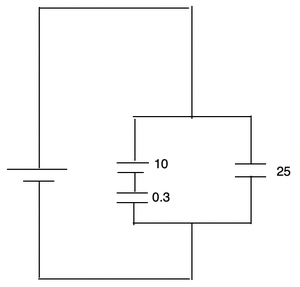The formula for parallel capacitance is given by,and the formula for series capacitance is given by,

C = C1 + C2 + C + ….

This is combination of both parallel and series capacitances.

substituting these values in the equation,

C1 = 10 μF  ,C = 0.3 μF⇒⇒⇒⇒ C = 0.29

C = C1 + C2

⇒ C = 0.29 + 2.5

⇒ C = 2.79

My Personal Notes arrow_drop_up
Related Tutorials